Next: Non-recirculating filter, second form Up: Elementary filters Previous: Elementary filters   Contents   Index

Elementary non-recirculating filter

The non-recirculating comb filter may be generalized to yield the design shown in Figure 8.7. This is the elementary non-recirculating filter, of the first form. Its single, complex-valued parametercontrols the complex gain of the delayed signal subtracted from the original one.To find its frequency response, as in Chapter 7 we feed the delay network a complex sinusoid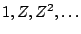whose frequency is. The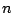th sample of the input is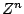and that of the output isso the transfer function isThis can be analyzed graphically as shown in Figure 8.8. The real numbersandare the magnitude and argument of the complex number:The gain of the filter is the distance from the pointto the pointin the complex plane. Analytically we can see this becauseGraphically, the numberis just the numberrotated backwards (clockwise) by the angular frequencyof the incoming sinusoid. The valueis the distance fromto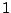in the complex plane, which is equal to the distance fromto.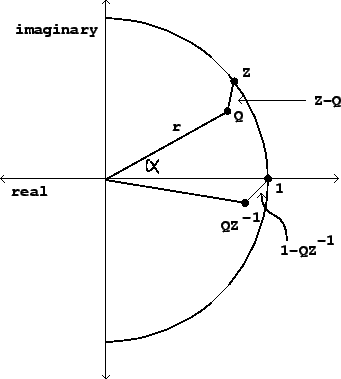As the frequency of the input sweeps from 0 to, the pointtravels couterclockwise around the unit circle. At the point where, the distance is at a minimum, equal to. The maximum occurs whichis at the opposite point of the circle. Figure 8.9 shows the transfer function for three different values of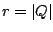.Next: Non-recirculating filter, second form Up: Elementary filters Previous: Elementary filters   Contents   Index
Miller Puckette 2006-12-30